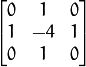#How I detect blurry images using OpenCV?

### Problem

I take a lot of picture and spend a good chunk of time discarding useless ones. I needed a better technique than the brute-force version I have now.

### Proposed solution

I was hoping if I could leverage some of the Laplacian kernel knowledge here to optimize things a bit. Just for starters, a Laplacian Kernel looks as follows.Laplacian Kernel

After some research, I found the following method: Variation of the Laplacian by Pech-Pacheco et al. in their 2000 ICPR paper, Diatom autofocusing in brightfield microscopy: a comparative study..

The method is simple and has a very sound reasoning. And can be implemented in only a single line of code:

``cv2.Laplacian(image, cv2.CV_64F).var()``

You simply take a single channel of an image (presumably grayscale) and convolve it with the Laplacian kernel shown above and then take the variance of the response.The variance can be used as a score to determine the blurriness of the image. The threshold for blurriness was a trial and error in my case.

### Why does this method work?

The reason this method works is due to the definition of the Laplacian operator itself, which is used to measure the 2nd derivative of an image. The Laplacian highlights regions of an image containing rapid intensity changes, much like the Sobel and Scharr operators. And, just like these operators, the Laplacian is often used for edge detection. The assumption here is that if an image contains high variance then there is a wide spread of responses, both edge-like and non-edge like, representative of a normal, in-focus image. But if there is very low variance, then there is a tiny spread of responses, indicating there are very little edges in the image. As we know, the more an image is blurred, the less edges there are.

### The trick

Obviously the trick here is setting the correct threshold which can be quite domain dependent. Too low of a threshold and you’ll incorrectly mark images as blurry when they are not. Too high of a threshold then images that are actually blurry will not be marked as blurry. This method tends to work best in environments where you can compute an acceptable focus measure range and then detect outliers.

### Sample Code

I am not going to dwelve into a lot of python code here but essentially this is all you have to do.

``````    import cv2
threshold = 110

gray = cv2.cvtColor(image, cv2.COLOR_BGR2GRAY)
score = cv2.Laplacian(image, cv2.CV_64F).var()

if score > threshold:
print "Not Blur"
else:
print "Blur"``````
Posted on February 10, 2018

← Next post    ·    Previous post →